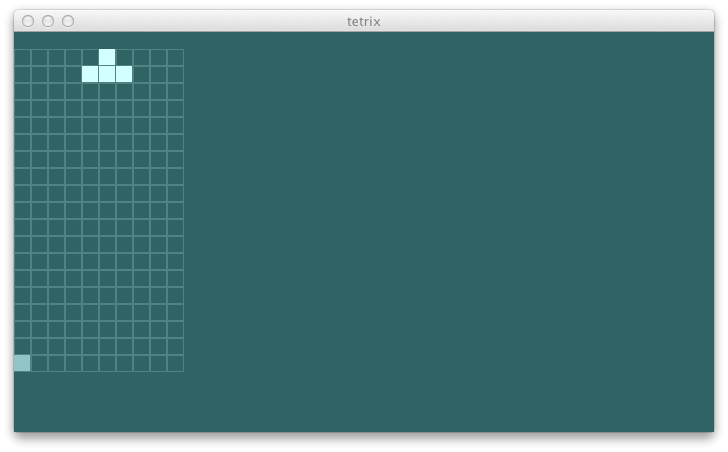# tetrix in Scala: day 1

Yesterday, we approximated the game state using `String`. Let's see how we can improve this.

### modeling the game

On screen there should be a 10x20 grid. I only want the current piece to be rendered in different color. We'll deal with the next piece window later. The different kinds of pieces can be represented using case objects:

```sealed trait PieceKind
case object IKind extends PieceKind
case object JKind extends PieceKind
case object LKind extends PieceKind
case object OKind extends PieceKind
case object SKind extends PieceKind
case object TKind extends PieceKind
case object ZKind extends PieceKind```

Individual blocks can be represented using a case class:

`case class Block(pos: (Int, Int), kind: PieceKind)`

Both the current piece and the grid can be presented using `Seq[Block]`.

`case class GameView(blocks: Seq[Block], gridSize: (Int, Int), current: Seq[Block])`

Now we can change the `AbstractUI` to return an instance of `GameView`.

```  def view: GameView =
GameView(
Seq(Block((5, 5), TKind), Block((6, 5), TKind), Block((7, 5), TKind), Block((6, 6), TKind), Block((0, 0), TKind)),
(10, 20),
Seq(Block((5, 5), TKind), Block((6, 5), TKind), Block((7, 5), TKind), Block((6, 6), TKind)))```

### drawing the game

This is enough information to start drawing the game better.

```  def onPaint(g: Graphics2D) {
val view = ui.view

def buildRect(pos: (Int, Int)): Rectangle =
new Rectangle(pos._1 * (blockSize + blockMargin),
(view.gridSize._2 - pos._2 - 1) * (blockSize + blockMargin),
blockSize, blockSize)
def drawEmptyGrid {
g setColor bluishLigherGray
for {
x <- 0 to view.gridSize._1 - 1
y <- 0 to view.gridSize._2 - 2
val pos = (x, y)
} g draw buildRect(pos)
}
def drawBlocks {
g setColor bluishEvenLigher
view.blocks foreach { b => g fill buildRect(b.pos) }
}
def drawCurrent {
g setColor bluishSilver
view.current foreach { b => g fill buildRect(b.pos) }
}
drawEmptyGrid
drawBlocks
drawCurrent
}```

Now that we have a way to visualize the game state, we should implement some moves.

### stage

We need a better way of representing the current piece besides a sequence of blocks. A `Piece` class should keep the current position in `(Double, Double)` and calculate the `current` from the local coordinate system.

```case class Piece(pos: (Double, Double), kind: PieceKind, locals: Seq[(Double, Double)]) {
def current: Seq[Block] =
locals map { case (x, y) =>
Block((math.floor(x + pos._1).toInt, math.floor(y + pos._2).toInt), kind)
}
}
case object Piece {
def apply(pos: (Double, Double), kind: PieceKind): Piece =
kind match {
case TKind => Piece(pos, kind, Seq((-1.0, 0.0), (0.0, 0.0), (1.0, 0.0), (0.0, 1.0)))
}
}```

This allows us to define `Stage` which enforces the physics within the game world.

```package com.eed3si9n.tetrix

class Stage(size: (Int, Int)) {
private[this] def dropOffPos = (size._1 / 2.0, size._2 - 3.0)
private[this] var currentPiece = Piece(dropOffPos, TKind)
private[this] var blocks = Block((0, 0), TKind) +: currentPiece.current
def view: GameView = GameView(blocks, size, currentPiece.current)
}```

To move the current piece, it is unloaded from the grid, moved to a new position, and then reloaded back in.

Here's the `moveBy` for `Piece` class:

```  def moveBy(delta: (Double, Double)): Piece =
copy(pos = (pos._1 + delta._1, pos._2 + delta._2))```

```class Stage(size: (Int, Int)) {
...

def moveLeft() = moveBy(-1.0, 0.0)
def moveRight() = moveBy(1.0, 0.0)
private[this] def moveBy(delta: (Double, Double)): this.type = {
val moved = currentPiece.moveBy(delta)
currentPiece = moved
this
}
private[this] def unload(p: Piece, bs: Seq[Block]): Seq[Block] = {
val currentPoss = p.current map {_.pos}
bs filterNot { currentPoss contains _.pos  }
}
private[this] def load(p: Piece, bs: Seq[Block]): Seq[Block] =
bs ++ p.current
}```

### wiring it up

Let's wire the stage up to the abstract UI:

```package com.eed3si9n.tetrix

class AbstractUI {
private[this] val stage = new Stage((10, 20))
def left() {
stage.moveLeft()
}
def right() {
stage.moveRight()
}
def up() {
}
def down() {
}
def space() {
}
def view: GameView = stage.view
}```

You should be able run swing UI and see the piece move.### specs2

Before we go any further we better have some specs. Testing UI-based games are not easy, but we've defined inputs and outputs in terms of data structure, so it's not that hard.

Add the latest specs2 to `library` project:

```  lazy val library = Project("library", file("library"),
settings = buildSettings ++ Seq(
libraryDependencies += "org.specs2" %% "specs2" % "1.12" % "test"
))```

Here's the specs for moving the current piece:

```import org.specs2._

class StageSpec extends Specification { def is = sequential  ^
"This is a specification to check Stage"                   ^
p^
"Moving to the left the current piece should"              ^
"""change the blocks in the view."""                     ! left1^
p^
"Moving to the right the current piece should"             ^
"""change the blocks in the view."""                     ! right1^
end

import com.eed3si9n.tetrix._
def stage = new Stage((10, 20))
def left1 =
stage.moveLeft().view.blocks map {_.pos} must contain(
(0, 0), (3, 17), (4, 17), (5, 17), (4, 18)
).inOrder
def right1 =
stage.moveRight().view.blocks map {_.pos} must contain(
(0, 0), (5, 17), (6, 17), (7, 17), (6, 18)
).inOrder
}```

### bdd

Now that we have a spec, let's try some "test first" coding. Given that the initial coordinate for the piece is `(5, 17)`, it takes four `moveLeft`s to hit the wall. The subsequent `moveLeft` should be ignored.

Here's the spec for hitting the left wall:

```  "Moving to the left the current piece should"              ^
"""change the blocks in the view,"""                     ! left1^
"""as long as it doesn't hit the wall"""                 ! leftWall1^

...

def leftWall1 =
stage.moveLeft().moveLeft().moveLeft().moveLeft().moveLeft().
view.blocks map {_.pos} must contain(
(0, 0), (0, 17), (1, 17), (2, 17), (1, 18)
).inOrder```

As expected, this test fails:

```[info] Moving to the left the current piece should
[info] + change the blocks in the view,
[error] x as long as it doesn't hit the wall
[error]    '(0,0), (-1,17), (0,17), (1,17), (0,18)' doesn't contain in order '(0,0), (0,17), (1,17), (2,17), (1,18)' (StageSpec.scala:8)```

We'll get back to this tomorrow.

```\$ git fetch origin
\$ git co day1 -b try/day1
\$ sbt "project swing" run```